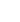15min:
LINE STRENGTHS IN THE FORM OF EINSTEIN A COEFFICIENTS AND OSCILLATOR STRENGTHS OF THE A2-X2+ (RED) AND B2+-X2+ (VIOLET) SYSTEMS OF CN.

R. S. RAM AND J. S. A. BROOKE, Department of Chemistry, University of York, York, YO10 5DD, UK; G. LI, Harvard-Smithsonian Center for Astrophysics, Cambridge, MA 02138, USA; D. W. SCHWENKE, NASA Ames Research Center, Moffett Field, CA 94035-1000, USA; P. F. BERNATH, Department of Chemistry and Biochemistry, Old Dominion University, Norfolk, VA 23529, USA.

Line strengths have been calculated in the form of Einstein A coefficients and oscillator strengths ( f -values) for a large number of bands of the A2-X2+ and B2+-X2+ transitions (with v =0-22 of A2, v =0-15 of B2+ and v =0-15 of X2+) of CN using Western's PGOPHER program. The potential energy curves of the three electronic states calculated using the experimental spectroscopic constants in an RKR program. The potential turning points of the three states and the transition dipole moments of two transitions calculated from high level ab initio calculations, were used in Le Roy's LEVEL program to produce rotation-less transition dipole moment matrix elements for a large number of bands. These transition dipole moments were then used in PGOPHER to generate a line list containing the observed and calculated wavenumbers, Einstein A coefficients and f -values. The Einstein A coefficients have been used to compute the radiative lifetimes of several vibrational levels of the A2and B2+ states. The computed values for some lower vibrational levels of the two states have been compared with values reported in previous experimental and theoretical studies.

A similar calculation on the line strengths of the A2-X2+ transition of CP is in progress and preliminary results will be presented.